# 深度学习第17讲：keras入门和快速上手指南keras 是一款使用纯 python 编写的神经网络 API，使用 keras 能够快速实现你的深度学习方案，所以 keras 有着为快速试验而生的美称。kerasTensorflowTheanoCNTK 为后端，意思就是 keras 的底层计算都是以以上这些框架为基础的，这使得 keras 能够专注于快速搭建起神经网络模型。

keras 搭建神经网络的基本流程



from keras.layers import Dense, Activation
 
model.add(Dense(units=64, input_dim=100))
 
model.add(Activation("relu"))
 
model.add(Dense(units=10))
 
model.add(Activation("softmax"))
 

model.compile(loss='categorical_crossentropy', optimizer='sgd', metrics=['accuracy'])

model.fit(x_train, y_train, epoche=5, batch_size=32)

loss_and_metrics = model.evaluate(x_test, y_test, batch_size=128)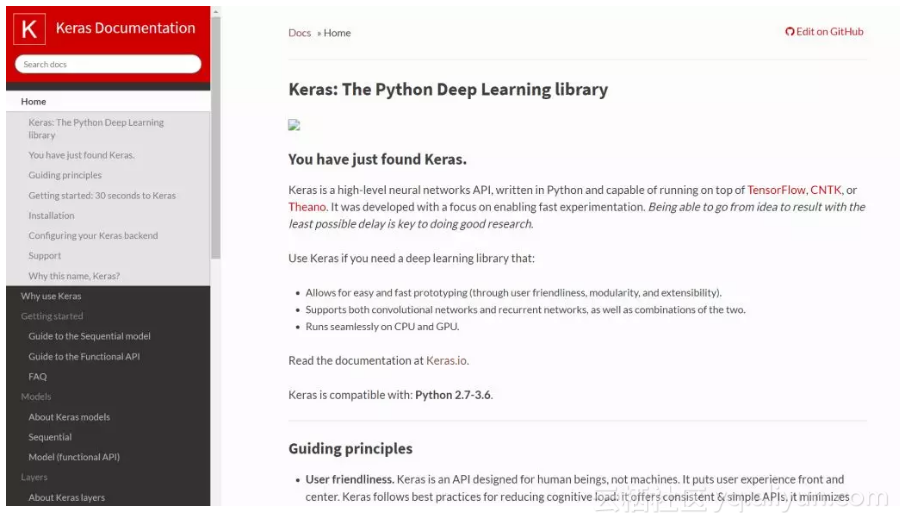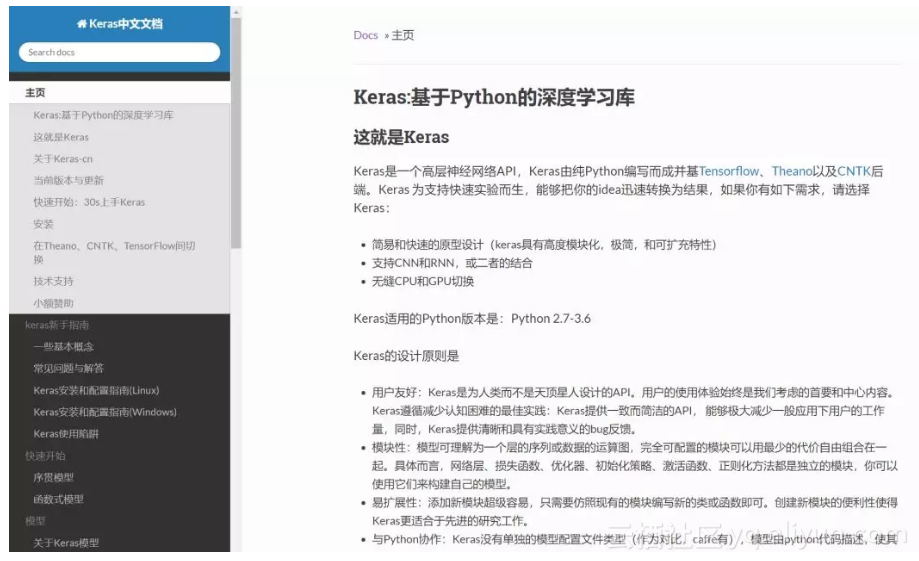keras 模拟数据例子



import keras
 
from keras.models import Sequential
 
from keras.layers import Dense, Dropout, Activation
 
from keras.optimizers import SGD
 

 
# Generate dummy data
 
import numpy as np
 
x_train = np.random.random((1000, 20))
 
y_train = keras.utils.to_categorical(np.random.randint(10, size=(1000, 1)), num_classes=10)
 
x_test = np.random.random((100, 20))
 
y_test = keras.utils.to_categorical(np.random.randint(10, size=(100, 1)), num_classes=10)
 
model = Sequential()
 
# Dense(64) is a fully-connected layer with 64 hidden units.
 
# in the first layer, you must specify the expected input data shape:# here, 20-dimensional vectors.
 
model.add(Dense(64, activation='relu', input_dim=20))
 
model.add(Dropout(0.5))
 
model.add(Dense(64, activation='relu'))
 
model.add(Dropout(0.5))
 
model.add(Dense(10, activation='softmax'))
 
sgd = SGD(lr=0.01, decay=1e-6, momentum=0.9, nesterov=True)
 
model.compile(loss='categorical_crossentropy',
 
 optimizer=sgd,
 
 metrics=['accuracy'])
 
model.fit(x_train, y_train,
 
 epochs=20,
 
 batch_size=128)
 
score = model.evaluate(x_test, y_test, batch_size=128)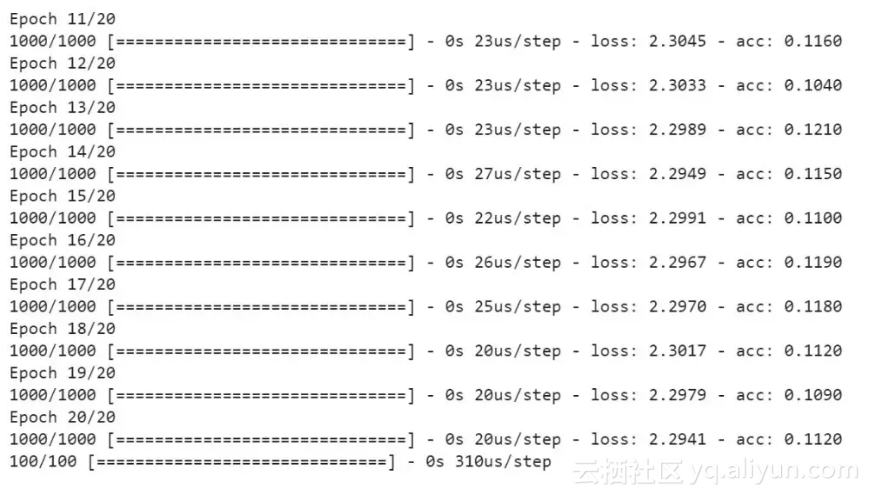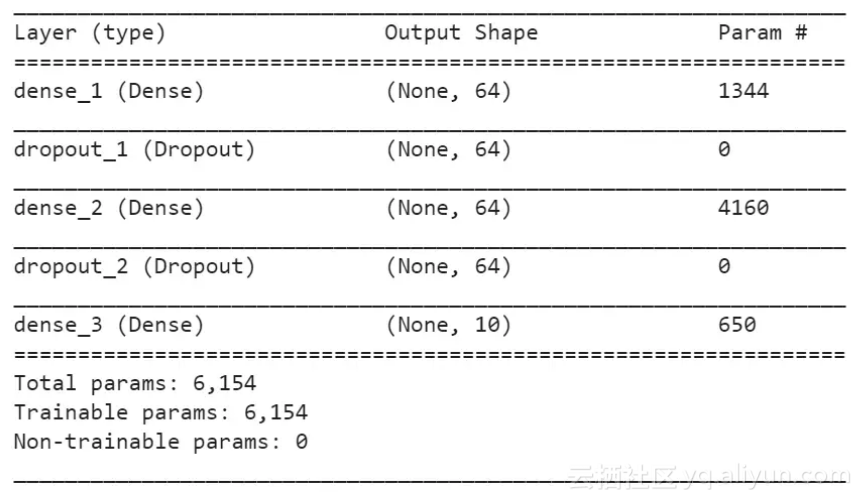import keras
 
from keras.layers import Conv2D, Dense, Dropout, Activation, MaxPooling2D, Flatten
 
from keras.models import Sequential
 
import numpy as np
 
import tensorflow as tf
 
from tensorflow.examples.tutorials.mnist import input_data
 

 
# define lenet5 model
 
def lenet5(X_train, y_train):
 
 model = Sequential()
 

 
 # first step: create sequential model and add layers
 
 model.add(Conv2D(6, (5, 5), strides=1, padding='valid',
 
 input_shape=(32, 32, 1), activation='relu'))
 
 model.add(MaxPooling2D(pool_size=(2, 2)))
 
 model.add(Conv2D(16, (5, 5), strides=1, activation='relu'))
 
 model.add(MaxPooling2D(pool_size=(2, 2)))
 
 model.add(Conv2D(1203, (5, 5), strides=1, activation='relu'))
 
 model.add(Flatten())
 
 model.add(Dense(84))
 
 model.add(Activation('relu'))
 
 model.add(Dropout(0.25))
 
 model.add(Dense(10))
 
 model.add(Activation('softmax'))
 
 # second step:compile the model
 
 model.compile(loss='categorical_crossentropy',
 
 optimizer='adam',
 
 metrics=['accuracy'])
 

 
 # third step: fit the model
 
 model.fit(X_train, y_train, batch_size=128, epochs=10)
 
 return model
 

 
# fourth step: evaluate the model
 
def eval_score(model, X_test, y_test):
 
 score = model.evaluate(X_test, y_test, batch_size=128)
 
 return score
 

 
if __name__ == '__main__':
 
 mnist = input_data.read_data_sets('MNIST_data', one_hot=True)
 
 sess = tf.InteractiveSession()
 X_train = mnist.train.images 
 X_train = tf.reshape(X_train, [-1, 28, 28, 1])
 
 X_train = tf.pad(X_train, [[0,0],[2,2],[2,2], [0,0]]).eval()
 
 y_train = mnist.train.labels
 X_test = mnist.test.images 
 X_test = tf.reshape(X_test, [-1, 28, 28, 1])
 
 X_test = tf.pad(X_test, [[0,0],[2,2],[2,2], [0,0]]).eval()
 
 y_test = mnist.test.labels
 
 model = lenet5(X_train, y_train)
 print(model.summary()) 
 score = eval_score(model, X_test, y_test)
 
 print(score)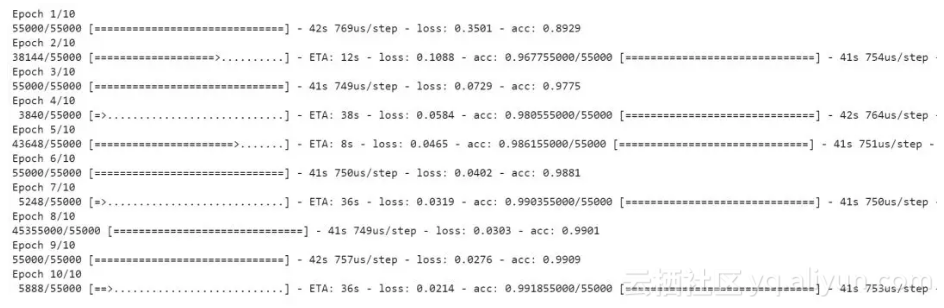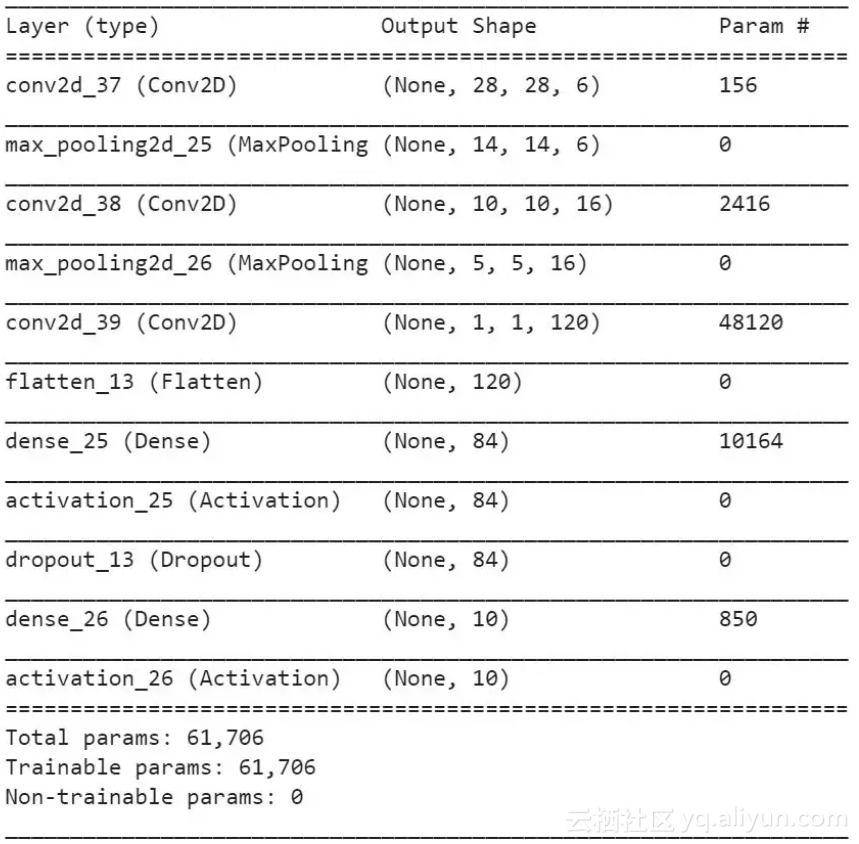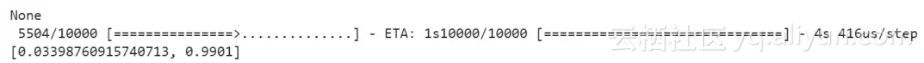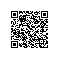Python爱好者使用钉钉扫一扫加入圈子
+ 订阅